Equivalent resistance of the given network between points A and B is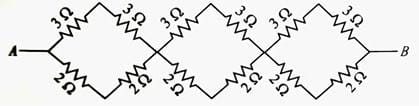• a)
31/5Ω
• b)
41/5Ω
• c)
36/5Ω
• d)
49/5Ω

### Related TestCiel Knowledge Jan 24, 2022In each segment of the combination 3Ω and 2Ω resistance are connected in series separately.
∴ R' = +3 = 6Ω and R'' = 2 + 2 = 4Ω
R' and R'' are connected in parallel
∴ For first segment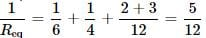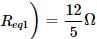Similarly for second and third segment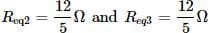Now segment is connected in series then the total resistance of combination is
Req = Req1 + Req2 + Req3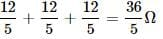In each segment of the combination 3Ω and 2Ω resistance are connected in series separately.∴ R = +3 = 6Ω and R = 2 + 2 = 4ΩR and R are connected in parallel∴ For first segmentSimilarly for second and third segmentNow segment is connected in series then the total resistance of combination isReq= Req1+ Req2+ Req3=
Do you know? How Toppers prepare for NEET Exam
With help of the best NEET teachers & toppers, We have prepared a guide for student who are preparing for NEET : 15 Steps to clear NEET Exam